# Tension on both sides of the rope is supposed to be the same?

• Monsterboy
The tension in the rope increases as the load (represented by W) is moved up.Just 14° more, when the rope adopts an angle of 84° respect to vertical, the value of the tension becomes 10W.As the angle tends to 90°, the tension tends to infinite.

#### Monsterboy

Homework Statement
A man has fallen into a ditch of width d and two of his friends are slowly pulling him out using a light rope and two fixed pulleys as shown in figure. Show that the force (assumed equal for both the friends) exerted by each friend on the road increases as the man moves up. Find the force when the man is at a depth h.
Relevant Equations
#T_1 cos \theta = T_2##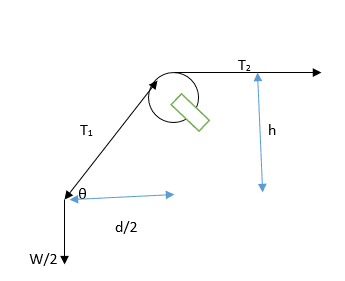The diagram represents one half of the system.
##W## is the weight of the man.
##T_1 cos \theta = T_2## --> The horizontal component of ##T_1 = T_2## right ?
##T_1 cos \theta = T_1 \frac {d}{\sqrt {4h^2 + d^2}} = T_2##
##T_1 sin \theta = \frac {W}{2}##
##T_1 = \frac {W}{2 sin \theta}##
##T_2 = \frac {W}{2 sin \theta} \frac {d}{\sqrt {4h^2 + d^2}}##

By taking ##sin \theta## as ##\frac {2h}{\sqrt{4h^2 + d^2}}##
##T_2 = \frac{Wd}{4h}##
By taking ##T_1 = \frac {T_2}{cos \theta}##
We get ##T_1 = \frac {W \sqrt{4h^2 + d^2}}{4h}##

But ##T_1## and ##T_2## are supposed to be the same right ?

Monsterboy said:
##T_1 cos \theta = T_2## --> The horizontal component of ##T_1 = T_2## right ?
How is the pulley staying in place?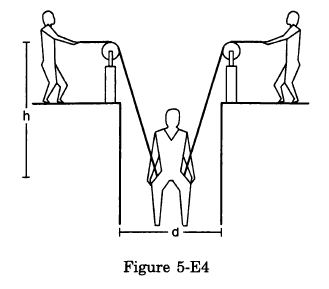•Monsterboy, Delta2 and sysprog
Thanks, @Lnewqban, I was wondering what "the diagram represents one half of the system" meant.

•Lnewqban
haruspex said:
How is the pulley staying in place?
The pulley is fixed in its position. Lnewqban's image is accurate.

Monsterboy said:
The pulley is fixed in its position. Lnewqban's image is accurate.
Right, so what might the forces on that look like?
Note that this is in relation to your equation that I quoted in post #2

haruspex said:
Right, so what might the forces on that look like?
Note that this is in relation to your equation that I quoted in post #2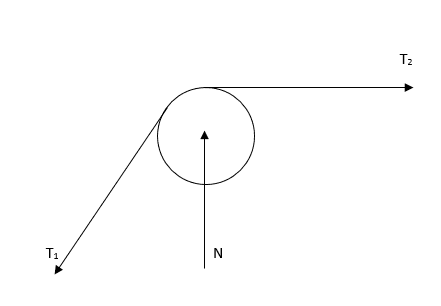The free body diagram of one pulley should look like above, the other one will be a mirror image.

The tension ## T_2 ## is created by the man's friends pulling the rope.
## T_1 ## has a vertical and a horizontal component. The vertical component (downwards) is balanced by the normal reaction of the pulley (upwards). The horizontal component is balanced by ## T_2 ## right ?

Monsterboy said:
View attachment 296083
The free body diagram of one pulley should look like above, the other one will be a mirror image.

The tension ## T_2 ## is created by the man's friends pulling the rope.
## T_1 ## has a vertical and a horizontal component. The vertical component (downwards) is balanced by the normal reaction of the pulley (upwards). The horizontal component is balanced by ## T_2 ## right ?
No. You are assuming there is no horizontal component to the support force on the pulley, and as a result deducing that the tensions are unequal. Since we know the tensions must be equal (consider torque balance on the pulley), your assumption must be wrong.

•Monsterboy
Monsterboy said:
View attachment 296083
The free body diagram of one pulley should look like above, the other one will be a mirror image.

The tension ## T_2 ## is created by the man's friends pulling the rope.
## T_1 ## has a vertical and a horizontal component. The vertical component (downwards) is balanced by the normal reaction of the pulley (upwards). The horizontal component is balanced by ## T_2 ## right ?
You can read the approximate direction in which the support force on the pulley must point by looking carefully at the diagram.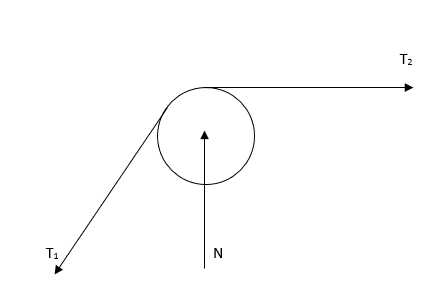The two tensions form an angle. The angle bisector (pointed up and to the left) is the direction the net force from the pulley on the cord must point.

•Monsterboy and Lnewqban
Monsterboy said:
View attachment 296083
The free body diagram of one pulley should look like above, the other one will be a mirror image.
These pulleys shown in the picture are hanging and therefore, are free to adopt the most natural angle.
The straight line formed between the axis of each pulley and its hanging point coincides with the direction of the reactive force keeping them from moving.
That force and the two tensions make a perfect symmetry.

The representation of force N in your diagram can't be vertical.
It would be only in the case in which both tensions are also vertical (not this case) or almost horizontal (when the rescued man is all the way up).

The angle of the direction of that reactive force on the fixed support of the pulley should be changing as the man is pulled up.
T1 and T2 should have the same magnitude.
A graphic vectors addition of T1 and T2 would help you visualize the direction and magnitude of that reactive force.Last edited:
•Monsterboy and sysprog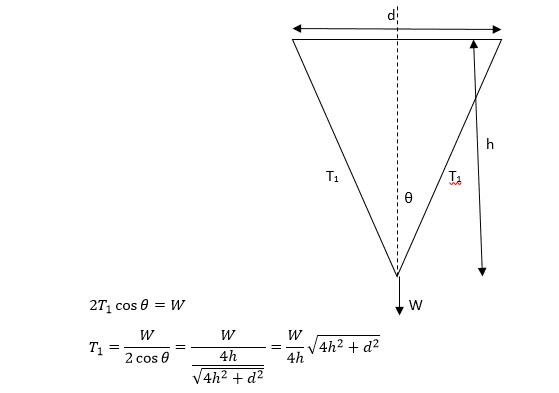I got the answer for the tension.

•sysprog and Lnewqban
Monsterboy said:
...
I got the answer for the tension.
The attached diagram has been drawn at scale and shows how the tension in the rope increases as the load (which weight has been represented as W) is moved up.
Note that when the rope adopts an angle of 60° respect to vertical, the value of the tension becomes 2W.
Just 14° more, when the rope adopts an angle of 84° respect to vertical, the value of the tension becomes 10W.
As the angle tends to 90°, the tension tends to infinite.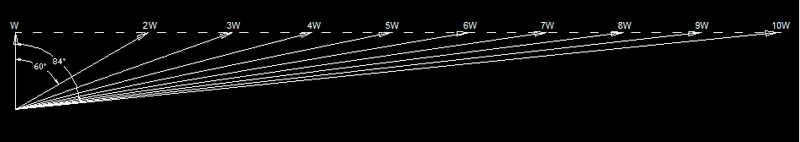Last edited:
•Monsterboy